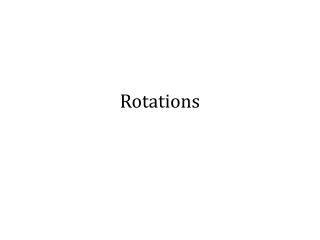# Rotations - PowerPoint PPT PresentationDownload PresentationRotations

RotationsDownload Presentation## Rotations

- - - - - - - - - - - - - - - - - - - - - - - - - - - E N D - - - - - - - - - - - - - - - - - - - - - - - - - - -
##### Presentation Transcript

1. Rotations

2. A rotation • turns all points of a figure around a point called the center of rotation • the angle of rotation tells us the number of degrees through which points rotate around the center of rotation

3. Properties of rotations A rotation is a transformation about a point P such that • every point and its image are the same distance from P and • all angles with vertex P formed by a point and its image have the same measure

4. Notation • The notation RP, m (A) = A’ says that the image of point A after a rotation of m degrees about point P is A’.

5. Rules for rotations in a coordinate plane rotation of 90°: (x,y)  (-y,x) rotation of 180°: (x,y)  (-x,-y) rotation of 270°: (x,y)  (y,-x) A positive angle of rotation turns the figure counterclockwise, and a negative angle of rotation turns the figure in a clockwise direction. Unless stated otherwise, rotations are counterclockwise.